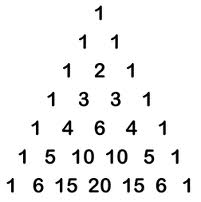# Binomial theorem

Expanding a binomial expression that has been raised to some large power could be troublesome; one way to solve it is to use the binomial theorem:

$(x+y)^{n}=1x^{n}y^{0}+\frac{n}{1}(x^{n-1}y^{1})+\frac{n(n-1)}{1\cdot 2}(x^{n-2}y^{2})+$

$+\frac{n(n-1)(n-2)}{1\cdot 2\cdot 3}(x^{n-3}y^{3})+...+1x^{0}y^{n}$

The expansion will have n+1 terms, there is always a symmetry in the coefficients in front of the terms.

Example

Expand the following binomial expression using the binomial theorem

$(x+y)^{4}$

The expansion will have five terms, there is always a symmetry in the coefficients in front of the terms. We use the binomial theorem to expand our binomial:

$(x+y)^{4}=1x^{4}y^{0}+\frac{4}{1}(x^{4-1}y^{1})+\frac{4(4-1)}{1\cdot 2}(x^{4-2}y^{2})+$

$+\frac{4\cdot 3\cdot 2}{1\cdot 2\cdot 3}(x^{4-3}y^{3})+\frac{4\cdot3\cdot2\cdot1}{1\cdot2\cdot3\cdot4}x^{0}y^{4}$

We simplify our expression and gets the following:

$(x+y)^{4}=x^{4}+4x^{3}y+6x^{2}y^{2}+4xy^{3}+y^{4}$

Note that the coefficients in front of our terms are 1, 4, 6, 4, 1. We could have found the first three coefficients and then used this symmetry to find the last two. Also note that the powers of x and y are reversed.

The coefficients in front of our terms could be found in an easier way - using Pascal´s triangle.

First study the following example

$(x+y)^{0}=\; \; \; \; \;\; \; \; \; \; \; \; \; \; \; \; \; {\color{green} 1}x^{0}y^{0}\\ (x+y)^{1}=\; \; \; \; \; \; \; \; \; \; \; {\color{green} 1}x^{1}y^{0}+{\color{green} 1}x^{0}y^{1}\\ (x+y)^{2}=\; \; \; \; \; {\color{green} 1}x^{2}y^{0}+{\color{green} 2}x^{1}y^{1}+{\color{green} 1}x^{0}y^{2}$

$(x+y)^{3}={\color{green} 1}x^{3}y^{0}+{\color{green} 3}x^{2}y^{1}+{\color{green} 3}x^{1}y^{2}+{\color{green} 1}x^{0}y^{3}$

The coefficients (in green) form a triangle called Pascal´s triangle and this is used in order to expand a binomial expression that has been raised to a large power. The following video lesson shows how Pascal´s triangle is created:## Video lesson

Example

Expand the following binomial expression using Pascal´s triangle

$(x+y)^{4}$

First we write up our factors

$x^{4}\;\; \; x^{3}y\; \; \; x^{2}y^{2}\; \; \; xy^{3}\; \; \; y^{4}$

Then we plug in our coefficients from Pascal´s triangle for a binomial expression powered to 4 which are 1, 4, 6, 4, 1:

$x^{4}+4 x^{3}y+6 x^{2}y^{2}+4 xy^{3}+ y^{4}$

and our expression is expanded.